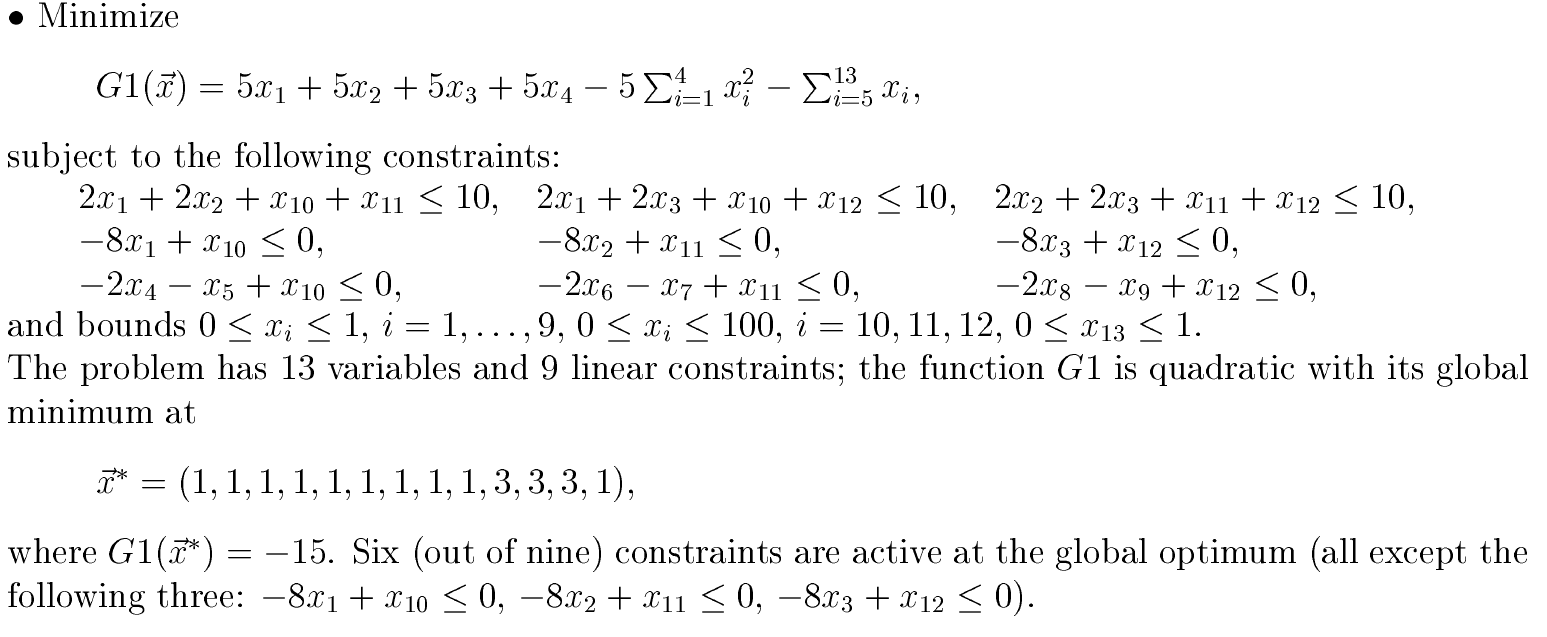# (Constrained) Numerical Optimization Problem (NOP)

A general constrained numerical optimization problem (NOP) may be written as follows:$\begin{array}{rcll} \min &~& f(\mathbf{x}) & \\ \mathrm{subject~to} &~& g_i(\mathbf{x}) =\in [c_i, d_i] &\text{for } i=1,\ldots,n \end{array}$

where$f(\mathbf{x})$ is the objective function and each$g(\mathbf{x})$ is a constraint function to be satisfied, and$c$ and$d$ are constants. Each function can be nonlinear and non-smooth. If there is no constraint (i.e.,$n=0$), then the problem becomes an unconstrained optimization problem.

NOP Instance Implementation [in DEPSO or SCO]

To implement your own problem instance, you need create a JAVA source file, normally placed in the directory problem/unconstrained (if the problem has no constraint) or problem/constrained (if the problem has constraints).

Implementation Tips: 1) all the variable bounds must be specified; 2)  any equality constraint should be relaxed by a small tolerance value (e.g., ε=1E-4 for problem.constrained.Michalewicz_G3); and 3) problem.ProblemEncoder and problem.UnconstrainedProblemEncoder are the parental classes of all constrained (e.g., problem.constrained.Michalewicz_G1) and unconstrained (e.g., problem.unconstrained.GoldsteinPrice) problems, respectively.

We use Michalewicz’s G1 problem as an example to explain the steps to implement an instance in JAVA. Here is the mathematical form:There are detailed comments in src/problem/constrained/Michalewicz_G1.java. It should be easy to figure out the implementation details.

Compiling Tips: Type “ant” to build, and the output file will be dist/depso.jar. You can also import the project into Eclipse IDE.

Testing Tips: There is a tool to calculate the output responses of the problem based on the input values. The tool has a main class problem.Simulator. It has two parameters, one is the function, and the second is the input values (separated by “,”). An example is:

\$ java -cp dist/depso.jar problem.Simulator problem.constrained.Michalewicz_G1 "1,1,1,1,1,1,1,1,1,3,3,3,1"

Posted on: Feb 15, 2018

Category : Code